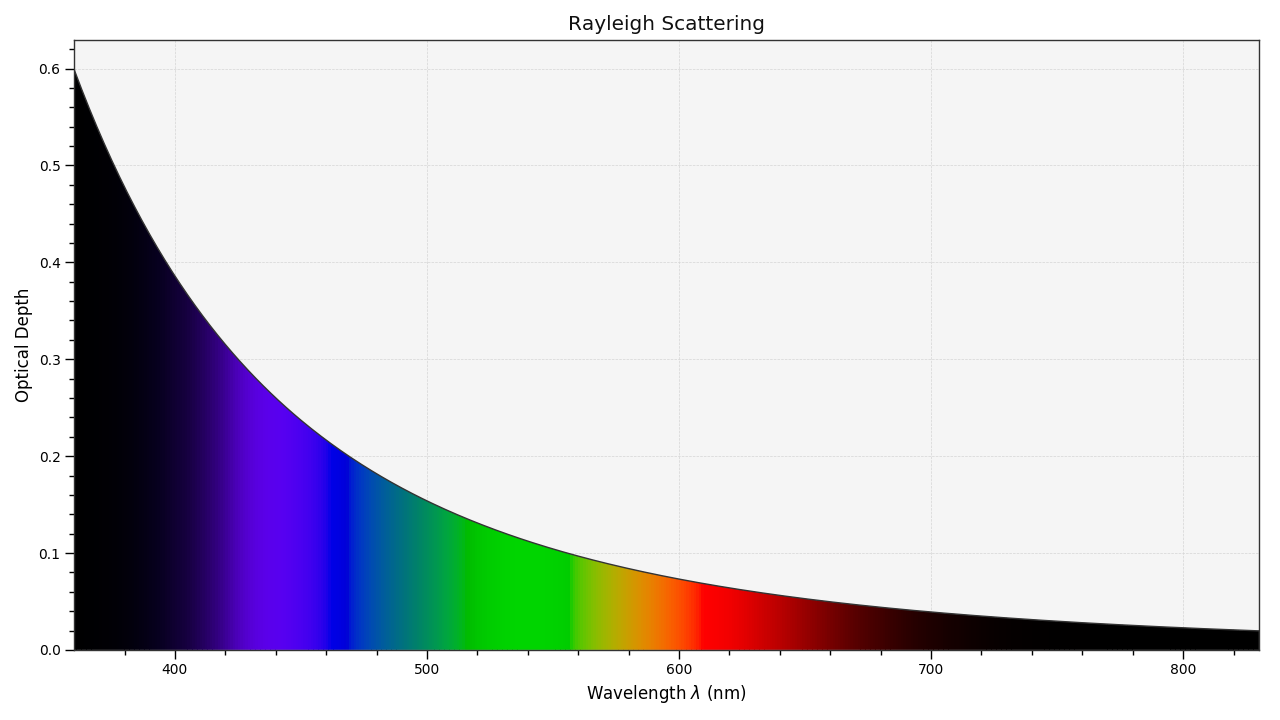# colour.plotting.plot_single_sd_rayleigh_scattering¶

colour.plotting.plot_single_sd_rayleigh_scattering(CO2_concentration=300, temperature=288.15, pressure=101325, latitude=0, altitude=0, cmfs='CIE 1931 2 Degree Standard Observer', **kwargs)[source]

Plots a single Rayleigh scattering spectral distribution.

Parameters
• CO2_concentration (numeric, optional) – $$CO_2$$ concentration in parts per million (ppm).

• temperature (numeric, optional) – Air temperature $$T[K]$$ in kelvin degrees.

• pressure (numeric) – Surface pressure $$P$$ of the measurement site.

• latitude (numeric, optional) – Latitude of the site in degrees.

• altitude (numeric, optional) – Altitude of the site in meters.

• cmfs (unicode, optional) – Standard observer colour matching functions.

Other Parameters
Returns

Current figure and axes.

Return type

tuple

Examples

>>> plot_single_sd_rayleigh_scattering()
(<Figure size ... with 1 Axes>, <matplotlib.axes._subplots.AxesSubplot object at 0x...>)